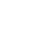# Vectors in Rust

In this lesson you'll learn about `Vec<T>`, or Vectors. Vectors are like Arrays, a collection of values of the same type, but as opposed to Arrays, Vectors can change in size.Anthony Albertorio
~ 11 months ago

For some reason, it possible to declare and initialize the same variable with different types. The compiler doesn't throw an error.

``````let numbers = "323";
let numbers = [1, 2, 3, 4];
let numbers = vec![1, 2, 3, 4];``````

Why is that?Anthony Albertorio
~ 11 months ago

For some reason, it possible to declare and initialize the same variable with different types. The compiler doesn't throw an error.

``````let numbers = [1, 2, 3, 4];
let numbers = vec![1, 2, 3, 4];
let numbers = "323";

println!("{}", numbers);``````

Why is that?

If I declare it this way, the compiler would throw an error related to `println!()` Am I just overriding the value of the variable?

``````let numbers = "323";
let numbers = [1, 2, 3, 4];
let numbers = vec![1, 2, 3, 4];

println!("{}", numbers);``````Pascal Prechtinstructor
~ 11 months ago

Hey Anthony!

For some reason, it possible to declare and initialize the same variable with different types. The compiler doesn't throw an error

Yes this is possible because you're essentially re-initializing the variable `numbers` here. It wouldn't work if you'd assign a new value (without putting a `let` in front of each statement).

If I declare it this way, the compiler would throw an error related to println!() Am I just overriding the value of the variable?

So for clarity's sake (and other readers), the error you're running into is:

10 | println!("{}", numbers); . | ^^^^^^^ `std::vec::Vec<{integer}>` cannot be formatted with the default formatter

What the compiler tells you year is that you're trying to print the vector `vec![1, 2, 3, 4]` with the default formatter (`{}`). Unfortunately, `Vec<{integer}>` doesn't implement the trait for this particular formatter, hence the error.

However, you might have guessed by now that there are other formatters. One of the is the debug formatter (`{:?}`), which does understand how to print a vector of your type.

So if you change the line to

``println!("{:?}", numbers);``

You should get the desired output. Here's a playground to verify this: https://play.rust-lang.org/?version=stable&mode=debug&edition=2018&gist=4f59ffeea319e96c46f6dd64a8b518f6

Notice that the only reason the order of how you re-initialize your variable matters here, is because the last value happens to be the vector. So whether you put `let numbers = "323"` at the top or remove it entirely, won't make a difference. The moment you try to print `numbers`, it's a `Vec<T>`.

Hope this makes sense!